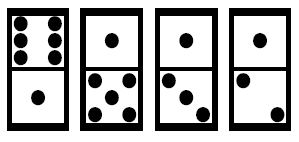Online JudgeProblem SetAuthorsOnline ContestsUser
Web Board
Home Page
F.A.Qs
Statistical Charts
Problems
Submit Problem
Online Status
Prob.ID:
Register
Update your info
Authors ranklist
Current Contest
Past Contests
Scheduled Contests
Award Contest
 User ID: Password:
Register
Language:
Dominoes
 Time Limit: 1000MS Memory Limit: 65536K Total Submissions: 7560 Accepted: 2499

Description

A domino is a flat, thumbsized tile, the face of which is divided into two squares, each left blank or bearing from one to six dots. There is a row of dominoes laid out on a table:The number of dots in the top line is 6+1+1+1=9 and the number of dots in the bottom line is 1+5+3+2=11. The gap between the top line and the bottom line is 2. The gap is the absolute value of difference between two sums.

Each domino can be turned by 180 degrees keeping its face always upwards.

What is the smallest number of turns needed to minimise the gap between the top line and the bottom line?

For the figure above it is sufficient to turn the last domino in the row in order to decrease the gap to 0. In this case the answer is 1.
Write a program that: computes the smallest number of turns needed to minimise the gap between the top line and the bottom line.

Input

The first line of the input contains an integer n, 1 <= n <= 1000. This is the number of dominoes laid out on the table.

Each of the next n lines contains two integers a, b separated by a single space, 0 <= a, b <= 6. The integers a and b written in the line i + 1 of the input file, 1 <= i <= 1000, are the numbers of dots on the i-th domino in the row, respectively, in the top line and in the bottom one.

Output

Output the smallest number of turns needed to minimise the gap between the top line and the bottom line.

Sample Input

```4
6 1
1 5
1 3
1 2
```

Sample Output

```1
```

Source

[Submit]   [Go Back]   [Status]   [Discuss]Home PageGo BackTo top

All Rights Reserved 2003-2013 Ying Fuchen,Xu Pengcheng,Xie Di
Any problem, Please Contact Administrator